# RD Sharma Solutions Ex-12.2, (Part -2), Heron's Formula, Class 9, Maths Class 9 Notes | EduRev

## Class 9 : RD Sharma Solutions Ex-12.2, (Part -2), Heron's Formula, Class 9, Maths Class 9 Notes | EduRev

The document RD Sharma Solutions Ex-12.2, (Part -2), Heron's Formula, Class 9, Maths Class 9 Notes | EduRev is a part of the Class 9 Course RD Sharma Solutions for Class 9 Mathematics.
All you need of Class 9 at this link: Class 9

Q8. Find the area of the quadrilateral ABCD in which AD = 24 cm, angle BAD = 90 and BCD forms an equilateral  triangle whose each side is equal to 26 cm. [Take √3 = 1.73]

Solution:

Given that, in a quadrilateral ABCD in which AD = 24 cm,

Angle BAD = 90∘

BCD is an equilateral triangle and the sides BC = CD = BD = 26 cm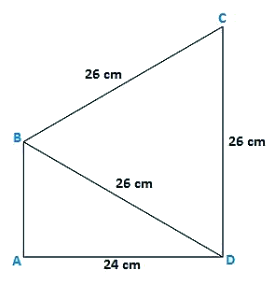In triangle BAD, by applying Pythagoras theorem,

BA2 = BD2−AD2

BA2 = 262+242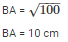Area of the triangle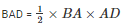Area of the triangle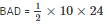Area of the triangle BAD = 120 cm2

Area of the equilateral triangle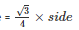Area of the equilateral triangle QRS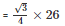Area of the equilateral triangle BCD = 292.37 cm2

Therefore, the area of quadrilateral ABCD = area of triangle BAD + area of the triangle BCD

The area of quadrilateral ABCD = 120 + 292.37

= 412.37 cm2

Q9.  Find the area of quadrilateral ABCD in which AB = 42 cm, BC = 21 cm, CD = 29 cm, DA = 34 cm and the diagonal BD = 20 cm.

Solution:

Given

AB = 42 cm, BC = 21 cm, CD = 29 cm, DA = 34 cm, and the diagonal

BD = 20 cm.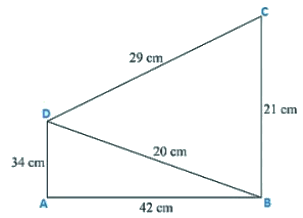Now, for the area of triangle ABD

Perimeter of triangle ABD 2s = AB + BD + DA

2s = 34 cm + 42 cm + 20 cm

s = 48 cm/p>

By using Heron’s Formula,

Area of the triangle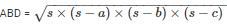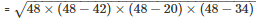= 336cm2

Now, for the area of triangle BCD

Perimeter of triangle BCD 2s = BC + CD + BD

2s = 29cm + 21cm + 20cm

s = 35 cm

By using Heron’s Formula,

Area of the triangle BCD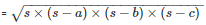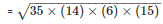= 210cm2

Therefore, Area of quadrilateral ABCD = Area of triangle ABD + Area of triangle BCD

Area of quadrilateral ABCD = 336 + 210

Area of quadrilateral ABCD = 546 cm2

Q10. Find the perimeter and the area of the quadrilateral ABCD in which AB = 17 cm, AD = 9 cm, CD = 12 cm, AC = 15 cm and angle ACB = 90.

Solution: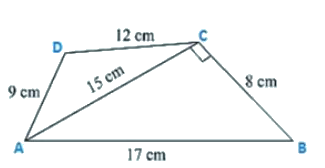Given are the sides of the quadrilateral ABCD in which

AB = 17 cm, AD = 9 cm, CD = 12 cm, AC = 15 cm and an angle ACB = 90

By using Pythagoras theorem

BC2 = AB2−AC2

BC2 = 172−152

BC = 8 cm

Now, area of triangle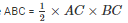area of triangle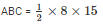area of triangle ABC = 60 cm2

Now, for the area of triangle ACD

Perimeter of triangle ACD 2s = AC + CD + AD

2s = 15 + 12 +9

s = 18 cm

By using Heron’s Formula,

Area of the triangle ACD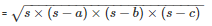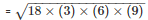= 54cm2

Area of quadrilateral ABCD = Area of triangle ABC + Area of triangle ACD

Area of quadrilateral ABCD = 60 cm2 + 54cm2

Area of quadrilateral ABCD = 114 cm2

Q11. The adjacent sides of a parallelogram ABCD measure 34 cm and 20 cm, and the diagonal AC measures 42 cm. Find the area of parallelogram.

Solution:

Given,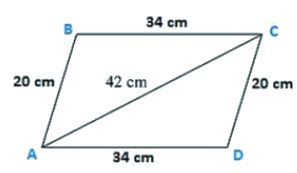The adjacent sides of a parallelogram ABCD measures 34 cm and 20 cm, and the diagonal AC measures 42 cm.

Area of the parallelogram = Area of triangle ADC + Area of triangle ABC

Note: Diagonal of a parallelogram divides into two congruent triangles

Therefore,

Area of the parallelogram = 2 × (Area of triangle ABC)

Now, for area of triangle ABC

Perimeter = 2s = AB + BC + CA

2s = 34 cm + 20 cm + 42 cm

s = 48 cm

By using Heron’s Formula,

Area of the triangle ABC =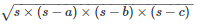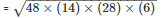= 336cm2

Therefore, area of parallelogram ABCD = 2 × (Area of triangle ABC)

Area of parallelogram = 2 × 336cm2

Area of parallelogram ABCD = 672 cm2

Q12. Find the area of the blades of the magnetic compass shown in figure given below.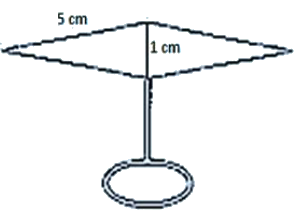Solution:

Area of the blades of magnetic compass = Area of triangle ADB + Area of triangle CDB

Now, for the area of triangle ADB

Perimeter = 2s = AD + DB + BA

2s = 5 cm + 1 cm + 5 cm

s = 5.5 cm

By using Heron’s Formula,

Area of the triangle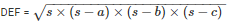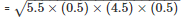= 2.49cm2

Also, area of triangle ADB = Area of triangle CDB

Therefore area of the blades of the magnetic compass = 2  × area of triangle ADB

Area of the blades of the magnetic compass = 2 ×2.49

Area of the blades of the magnetic compass = 4.98 cm2

Q13. A hand fan is made by sticking 10 equal size triangular strips of two different types of paper as shown in the figure. The dimensions of equal strips are 25 cm, 25 cm and 14 cm. Find the area of each type of paper needed to make the hand fan.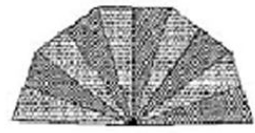Solution:

Given that,

The sides of AOB

AO = 25 cm

OB = 25 cm

BA = 14 cm

Area of each strip = Area of triangle AOB

Now, for the area of triangle AOB

Perimeter = AO + OB + BA

2s = 25 cm +25 cm + 14 cm

s = 32 cm

By using Heron’s Formula,

Area of the triangle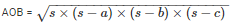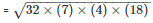= 168cm2

Also, area of each type of paper needed to make a fan = 5 ×  Area of triangle AOB

Area of each type of paper needed to make a fan = 5 × 168 cm2

Area of each type of paper needed to make a fan = 840cm2

Q14. A triangle and a parallelogram have the same base and the same area. If the sides of the triangle are 13 cm, 14 cm and 15 cm and the parallelogram stands on the base 14 cm, find the height of a parallelogram.

Solution:

The sides of the triangle DCE are

DC = 15 cm,

CE = 13 cm,

ED = 14 cm

Let the h be the height of parallelogram ABCD

Now, for the area of triangle DCE

Perimeter = DC + CE + ED

2s  = 15 cm + 13 cm + 14 cm

s = 21 cm

By using Heron’s Formula,

Area of the triangle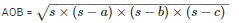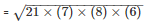= 84cm2

Also, area of triangle DCE = Area of parallelogram ABCD ⇒ 84cm2

24 × h  = 84cm2

h = 6 cm.

Offer running on EduRev: Apply code STAYHOME200 to get INR 200 off on our premium plan EduRev Infinity!

91 docs

,

,

,

,

,

,

,

,

,

,

,

,

,

,

,

,

,

,

,

,

,

,

,

,

,

,

,

,

,

,

,

,

,

;